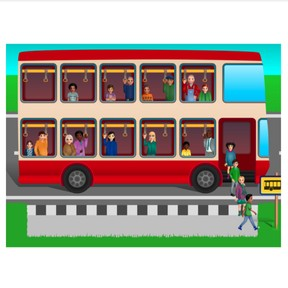On the bus: Subtraction to 20

# On the bus: Subtraction to 20

No account needed.8,000 schools use Gynzy92,000 teachers use Gynzy1,600,000 students use Gynzy

## General

Students learn to subtract numbers to 20 using the context of a bus ride.

1.OA.C

## Relevance

To learn to calculate well, it is important to be able to subtract numbers to 20. Using the bus ride as a context helps solidify the understanding of what happens in a subtraction problem which increases ease of calculation.

## Introduction

Show students the image of a bus driver and ask students if they have ever been on a busy bus and gotten off with lots of people at the same time. Where was this? This often happens at a concert, sporting event, or other large events. Ask students to count the passengers waiting for the bus in the next three images.

## Development

Using the three images, explain how subtraction on the bus work. The first image shows how many passengers are on the bus as it arrives. The second shows how many passengers exit the bus at the stop. The third image shows how many passengers are left on the bus as it leaves. You can determine this third image total by taking away the number of exiting passengers from the total number of passengers from the first image. Ask students to name which number is the first number of the subtraction problem (minuend) which is the second number of the subtraction problem (subtrahend) and what is the total, or difference. Tell students that they can check their work by adding their total (difference) and second number (subtrahend) together.
Next practice two more bus examples on the interactive whiteboard. Explain to students that the images will now stay the same- you can have all the information in just one image. Practice this with two bus examples and show students that they can also solve using the bus method even when they can't count the individual passengers, but are given numbers to represent the number of passengers on the bus. Practice with the students.

To check that students are able to subtract numbers to 20 using the context of a bus ride, you can ask the following questions:
- What are the steps to solving a subtraction problem on the bus?
- What does the first number in the subtraction problem represent?
- What does the second number in the subtraction problem represent?

## Guided practice

Students first work to match the given image with a subtraction problem. Then they are asked to solve a given subtraction problem with visual support. Students are then asked to subtract on the bus without being able to count the individual passengers but with numbers.

## Closing

Repeat the learning goal and ask students what the steps are to solving a subtraction problem on the bus. Ask students to determine how many passengers are still on the bus after some have stepped out of the bus. Ask students what the subtraction problem is for that image. Finally, ask students to work in pairs to create a subtraction problem on the bus for their partners. They draw a bus with passengers on the bus and waiting for the bus. They must use between 10-20 passengers. When they have created their subtraction problem on the bus they trade papers with their partner and solve the given subtraction problem.

## Teaching tips

Students who have difficulty with this learning goal can be supported by a clear breakdown of steps. First counting the total passengers on the bus, then counting how many passengers get off the bus, and finally counting how many passengers are still left on the bus. They can use blocks or other manipulatives to represent the passengers.

## Instruction materials

paper, pencils
optional: blocks or manipulatives

### The online teaching platform for interactive whiteboards and displays in schools

• Save time building lessons

• Manage the classroom more efficiently

• Increase student engagement